By Long Luo

# Intuition

We can use Recursion to solve this problem.

The key points of Recursion are $2$.

1. How to terminate the recursion: Returns when either $\texttt{l1}$ or $\texttt{l2}$ is $\texttt{null}$.

2. What to do in the process: if $\texttt{l1.val} \le \texttt{l2.val}$, then point $\texttt{l1.next}$ to the smaller of $\texttt{l1}$ and $\texttt{l2}$.

If $\texttt{l1.val} \le \texttt{l2.val}$, we can choose the smaller node, such as $\texttt{l1}$. However, the linked list is not reached the end, we will continue to compare.

Now we are compare $\texttt{l1.next}$ and $\texttt{l2}$. The $\texttt{l1.next}$ and $\texttt{l2}$ are processed in the recursive functions of the next layer.

We process such process and finally get the result.

# Image Explanation

You can get a intuition from below images.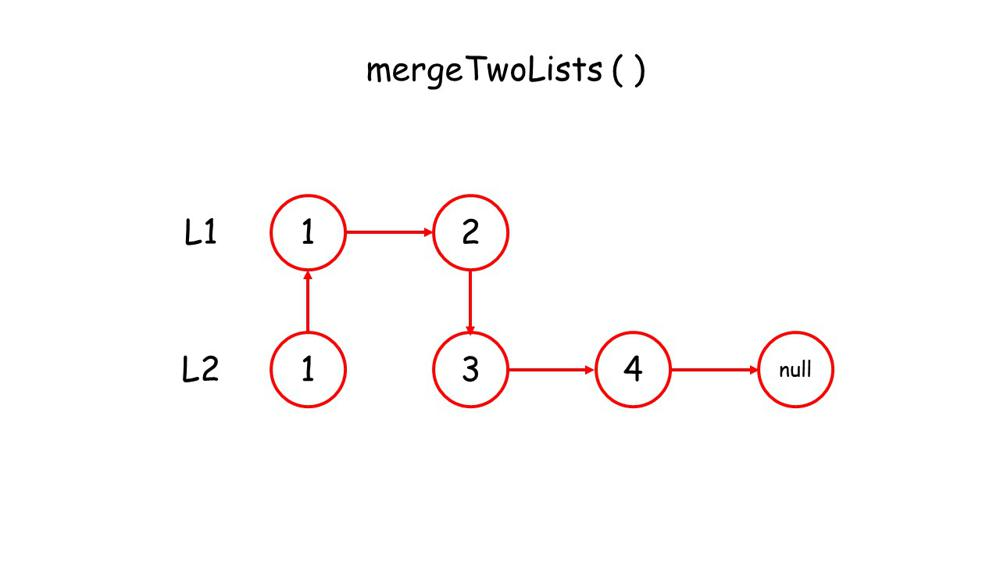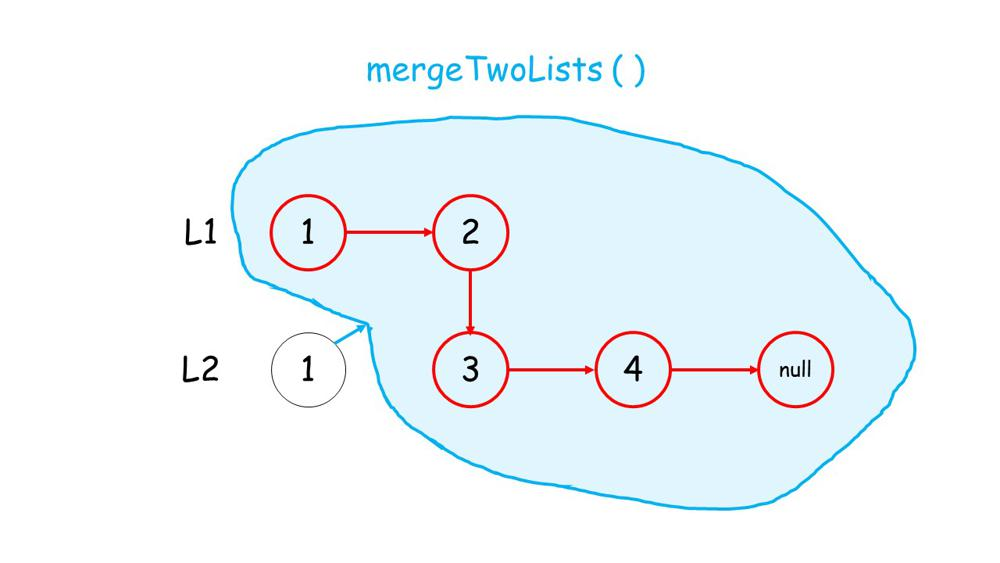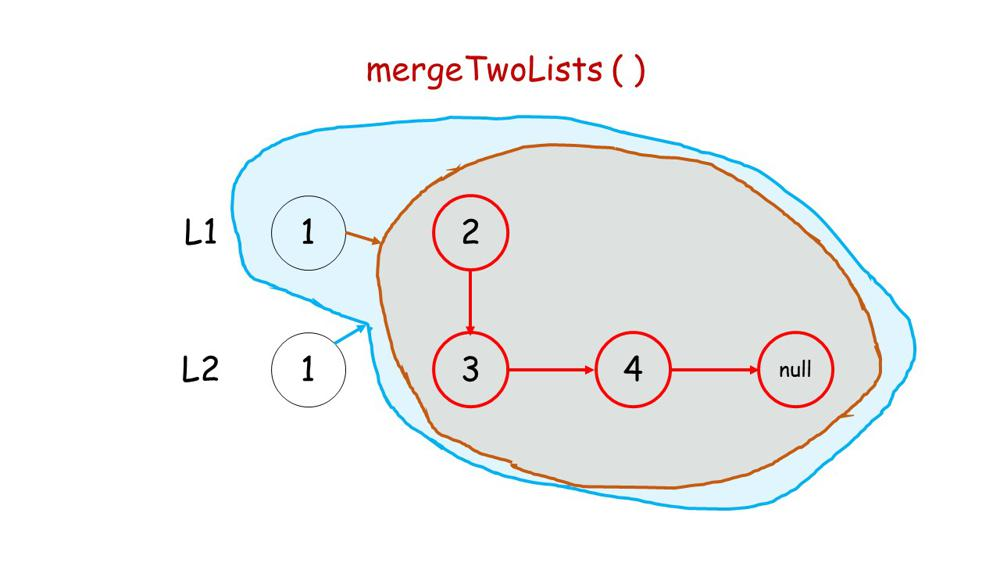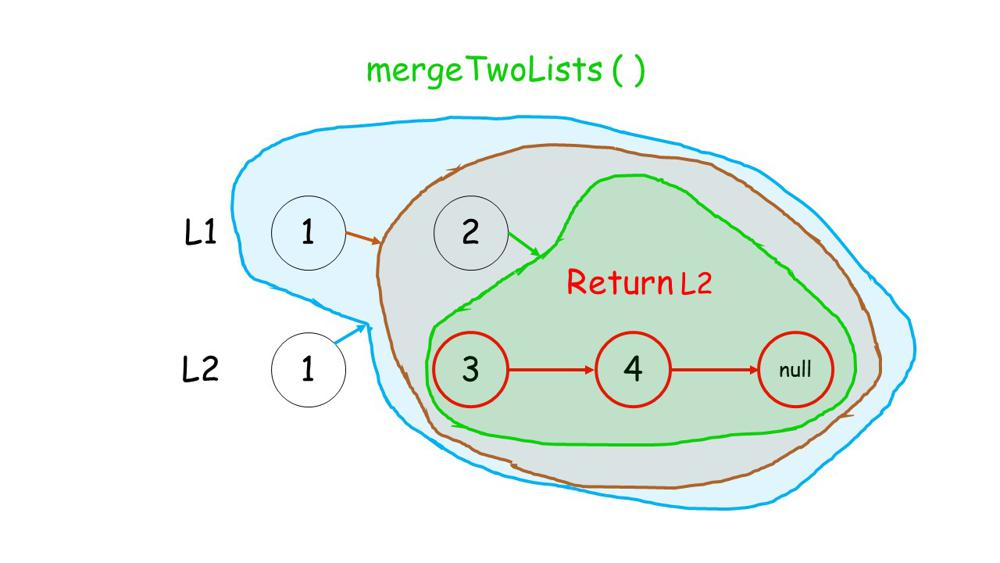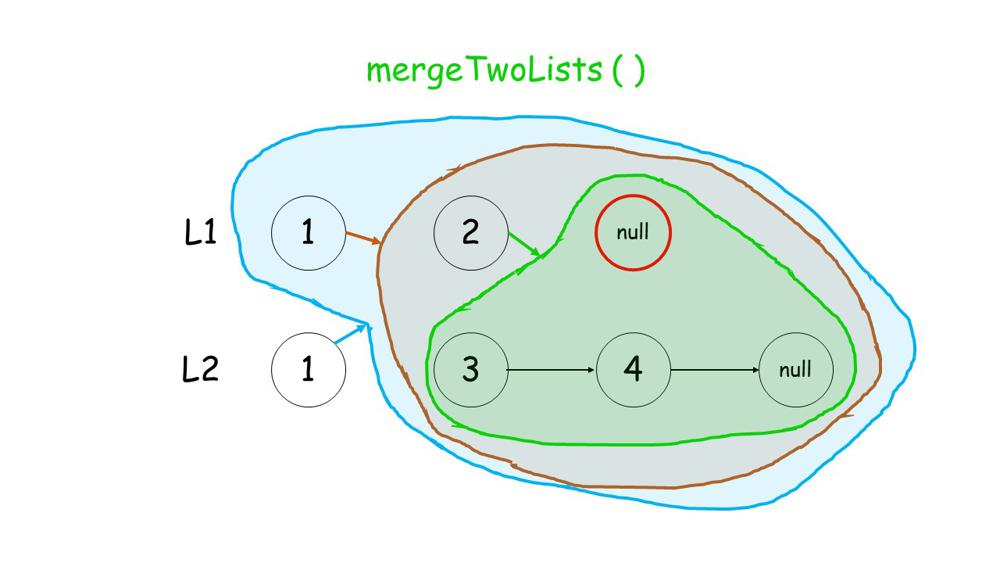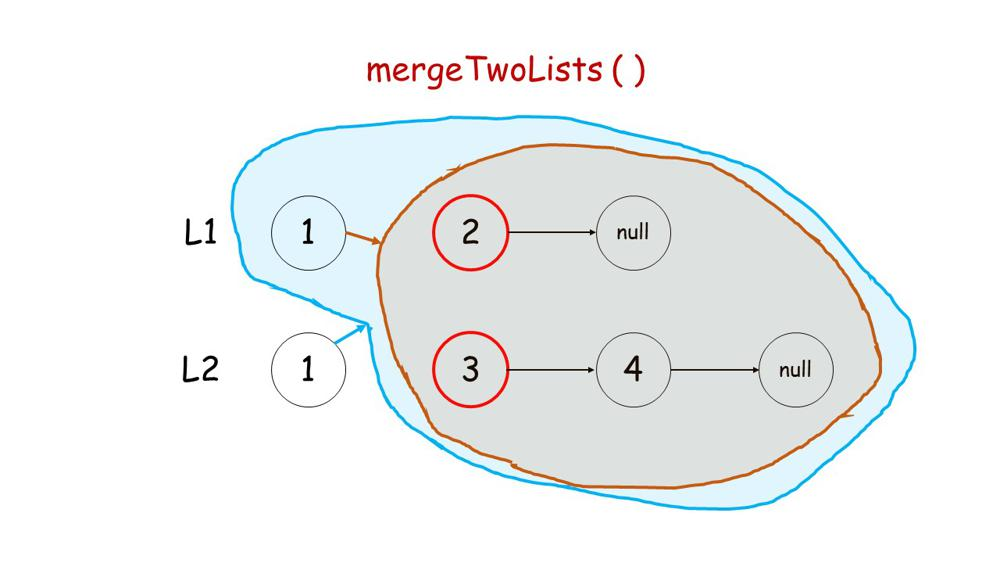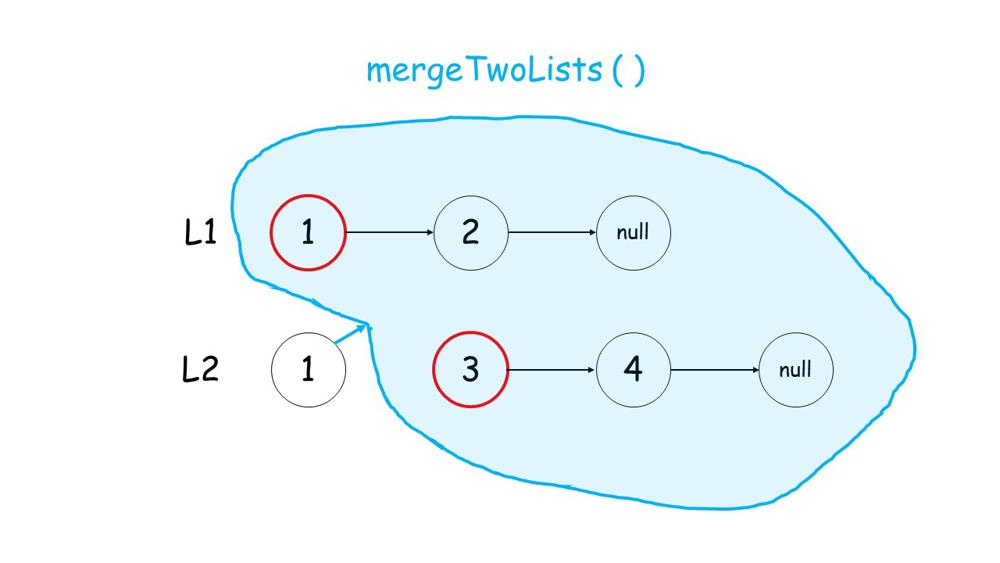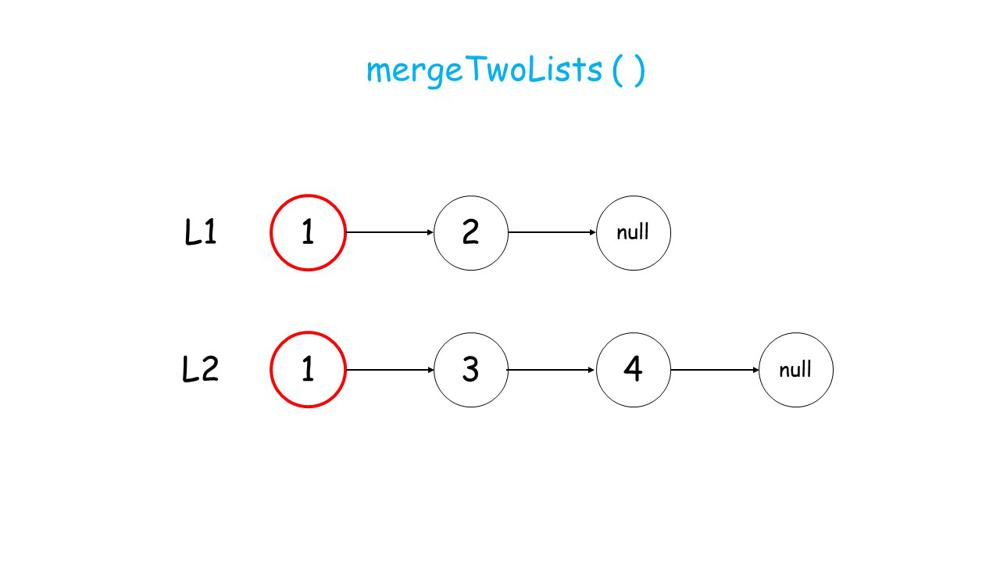## Analysis

• Time Complexity: $O(m + n)$.
• Space Complexity: $O(m + n)$.

All suggestions are welcome.
If you have any query or suggestion please comment below.
Please upvote👍 if you like💗 it. Thank you:-)

Explore More Leetcode Solutions. 😉😃💗